## Example Questions

← Previous 1

### Example Question #101 : Arithmetic

Simplify the following: (√(6) + √(3)) / √(3)

3√(2)

1

√(2) + 1

√(3)

√(2) + 1

Explanation:

Begin by multiplying top and bottom by √(3):

(√(18) + √(9)) / 3

Note the following:

√(9) = 3

√(18) = √(9 * 2) = √(9) * √(2) = 3 * √(2)

Therefore, the numerator is: 3 * √(2) + 3.  Factor out the common 3: 3 * (√(2) + 1)

Rewrite the whole fraction:

(3 * (√(2) + 1)) / 3

Simplfy by dividing cancelling the 3 common to numerator and denominator: √(2) + 1

### Example Question #1 : How To Simplify Square Roots

what is

√0.0000490

0.00007

0.07

0.007

7

49

0.007

Explanation:

easiest way to simplify: turn into scientific notation

√0.0000490= √4.9 X 10-5

finding the square root of an even exponent is easy, and 49 is  a perfect square, so we can write out an improper scientific notation:

√4.9 X 10-5√49 X 10-6

√49 = 7; √10-6 = 10-3 this is equivalent to raising 10-6 to the 1/2 power, in which case all that needs to be done is multiply the two exponents: 7 X 10-3= 0.007

### Example Question #102 : Arithmetic

Simplify: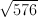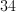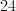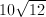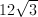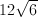Explanation:

In order to take the square root, divide 576 by 2.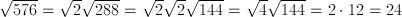### Example Question #1 : How To Simplify Square Roots

Simplify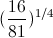.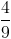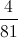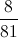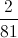Explanation: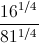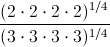### Example Question #1 : How To Simplify Square Roots

Simplfy the following radical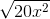.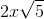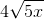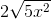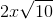Explanation:

You can rewrite the equation as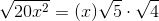.

This simplifies to.

### Example Question #1 : Simplifying Square Roots

Which of the following is equal to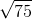?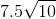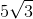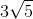Explanation:

√75 can be broken down to √25 * √3. Which simplifies to 5√3.

### Example Question #21 : Simplifying Square Roots

Simplify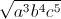.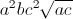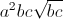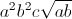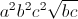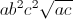Explanation:

Rewrite what is under the radical in terms of perfect squares: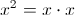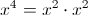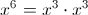Therefore,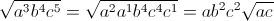.

### Example Question #1 : Simplifying Square Roots

What is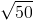?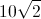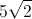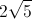Explanation:

We know that 25 is a factor of 50. The square root of 25 is 5. That leaves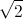which can not be simplified further.

### Example Question #2 : Simplifying Square Roots

Which of the following is equivalent to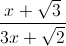?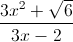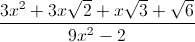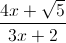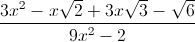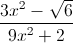Explanation:

Multiply by the conjugate and the use the formula for the difference of two squares: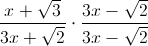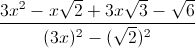### Example Question #10 : How To Simplify Square Roots

Which of the following is the most simplified form of: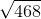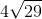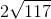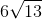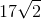Explanation:

First find all of the prime factors of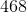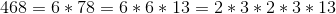So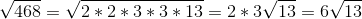← Previous 1

Tired of practice problems?

Try live online GRE prep today.